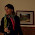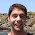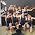## Wednesday, January 13, 2016

The connection between functional reactive programming and temporal logic is well known and studied. I want to describe how an FRP interface in Haskell based on temporal logic could look like. I should also cite these slides from Uni Muenchen and this paper.

### Inspiration from temporal logic

If we go to wikipedia we see that temporal logic has three basic unary operators called `Next`, `Globally` and `Finally` (abbreviated `X`, `G` and `F`). They are explained further down on that page. `Globally` and `Finally` translate to the familiar `Behavior` and `Event` types. `Next` is a type I have never seen in an FRP library before. It protects the future from access in the now, similar to `MomentIO` or `Now`.

In the equivalences section under Special temporal properties we see two interesting equivalences that we take to be our basic axioms:

``````G Φ ≡ Φ ∧ X(G Φ)
F Φ ≡ Φ ∨ X(F Φ)
``````

Translated to Haskell this is

``````data Behavior a = AndThen {
now :: a,
future :: Next (Behavior a) }

data Event a =
Occured a |
Later (Next (Event a))
``````

`Behavior` is Cofree instantiated with `Next` and `Event` is Free instantiated with `Next`.

We have to make one other assumption: that `Next` is an instance of `Applicative`. In this post for simplicity we define `Next` to be `IO`:

``````type Next = IO
``````

### Deriving temporal logic axioms

My search did not reveal what axioms temporal logic usually rests on. But temporal logic is a specific modal logic and as far as I understand modal logic axioms sometimes include (notation taken from wikipedia and explained below):

• N: p → □p
• K: □(p → q) → (□p → □q).
• T: □p → p
• 4: □p → □□p
• B: p → □◇p
• D: □p → ◇p
• 5: ◇p → ◇□p

Ok, this notation is different to what we saw earlier. The little box is what we called `G` and corresponds to `Behavior` and the little diamond is what we called `F` and corresponds to `Event`. Under this notation `X` (called `Next` in our interpretation) would be denoted with a little circle.

Axioms `N` and `K` are found in all modal logics while the others are more optional. `N` is called the Necessitation Rule, axiom `K` is called the Distribution Axiom and axiom `T` is called the Reflexivity Axiom. In Haskell they correspond to `pure`, `ap` and `extract`. Axiom `4` has no long name in logic but in Haskell it is called `duplicate`.

These axioms become theorems because they follow from our basic axioms. We can derive them using our basic axioms which is to say we can implement them using our data type definitions. For example a `Behavior` that is always the same is:

``````always :: a -> Behavior a
always a = a `AndThen` pure (always a)
``````

This corresponds to the `N` axiom. Implementations of some of the other axioms seem trivial and do not make sense as general purpose combinators.

### Implementing FRP functions

On the other hand we can easily implement functions known from FRP libraries. For example an event that never happens:

``````never :: Event a
never = Later (pure never)
``````

Or we can sample a behavior when an event occurs:

``````sample :: Event a -> Behavior b -> Event (a, b)
sample (Occured a) (AndThen b _) =
Occured (a, b)
sample (Later nextEvent) (AndThen _ nextBehavior) =
Later (liftA2 sample nextEvent nextBehavior)
``````

### A symmetric IO interface

If we have the ability to lift `IO` actions into `Next` we get a nice, symmetric IO interface similar to `fromPoll`, `reactimate` and `planNow`:

``````plan :: Event (Next a) -> Next (Event a)
plan (Occured next) =
fmap Occured next
plan (Later next) =
fmap (Later . plan) next

poll :: Behavior (Next a) -> Next (Behavior a)
poll behavior =
liftA2 AndThen
(now behavior)
(fmap poll (future behavior))
``````

Luckily in our case `Next` is `IO` and we can lift `IO` actions into `Next` with:

``````syncIO :: IO a -> Next a
syncIO = id
``````

To poll the current time using `getCurrentTime` we would write the following:

``````currentTime :: Next (Behavior UTCTime)
currentTime =
poll (always (syncIO getCurrentTime))
``````

We get an event that fires upon the next user input with:

``````nextLine :: Next (Event String)
nextLine =
plan (Occured (syncIO getLine))
``````

### Running Behaviors and Events

We can run an event until it occurs, performing all side effects while doing so:

``````runNext :: Next a -> IO a
runNext = id

runEvent :: Event a -> IO a
runEvent event = case event of
Occured a ->
return a
Later nextEvent -> do
event' <- runNext nextEvent
runEvent event'
``````

### An example application

Let’s finish with a small example application. It will keep asking the user for input, then print the user’s input and the current time. It will stop if the user enters `"exit"`. The parameters of this function are the event of the next user input and a behavior that carries the current time.

``````loop :: Event String -> Behavior UTCTime -> Event ()

loop (Occured "exit") _ = do
Occured ()

loop (Occured message) (AndThen time futureTime) =
Later (
syncIO (putStrLn (show time ++ ": " ++ message)) *>
liftA2 loop nextLine futureTime)

loop (Later nextEvent) (AndThen _ futureTime) =
Later (
liftA2 loop nextEvent futureTime)
``````

And finally we run this loop:

``````main :: IO ()
main = runEvent (Later (
liftA2 loop nextLine currentTime))
``````

Example interaction:

``````> hi
2016-01-13 11:44:58.059605 UTC: hi
> what's new
2016-01-13 11:45:00.178503 UTC: what's new
> exit
``````

In conclusion we have taken two temporal logic theorems as axioms and based an implementation of FRP on them. This implementation happens to be `Cofree` for behaviors and `Free` for events over a `Next` functor. In this post we used `IO` for `Next` but I have a feeling that the power of this approach lies in the fact that we can vary this functor.

1.hi,
>Axioms N and K are found in all modal logics while the others are more optional.
this is NOT true! N (as you have it) trivializes modal logic, (Box A<->A) when the much more usual axiom T (Box A-> A) is true. the rule for Necessitation is a real rule, cannot be made into an axiom. loads of work written about it, local consequences vs global consequences.

1.Hi, thank you for your reply, you are a absolutely right! I did not understand that N was a rule and not an axiom.

2.Fantastic!
I think there're some ideas shared by those(http://dlaing.org/cofun/) and yours.
Free as a DSL, Cofree as an Interpreter, Just like Event and Behavior.

3.I knew I was onto something when I thought of this*! Sadly I couldn't make it through any of the related papers, and hadn't even heard of Cofree

*this being a link between temporal logic and FRP

4.I knew I was onto something when I thought of this*! Sadly I couldn't make it through any of the related papers, and hadn't even heard of Cofree

*this being a link between temporal logic and FRP

5.This looks really cool - but how do you write filter for events with this (or at least what should the type signature be?).

1.In fact, can you ever have an `Event` with produces more than one event? I guess I'm coming from other FRP styles where `Event` is normally a stream of events, where as this seems to always terminate when the `Event` is `Occurred` as there's no way to get a continuation of the the stream once `Occurred` has happened?

2.You are right, in this formulation an event is a single occurence and a stream of events would be something else. I am not sure what.